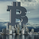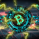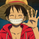TradingView
1682 views
This is a simple momentum indicator . It calculates the moving average of gains and losses. As you can see in the chart above, gains have been decreasing in each wave which is also captured by this indicator. Its closest relative is RSI but unlike RSI it is not range bound. Divergence on indicators that are not range bound are generally more reliable.

Recommended settings:
- EMA as moving average type.
- EMA length 27 is equal to RSI length 14 (2 x RSI length - 1). You can sync the length on the two indicators using that equation.
- hlc3 ( AKA typical price) as input source might be better than "close" as it captures more information. If you use hlc3 as a source, then change the chart type to line and set hlc3 as the source for identifying divergence.

PS: Let me know if you know any identical indicator. I may be re-inventing the wheel :)
Release Notes: - implemented the same fix as True RSI
- made the code a bit more clear
Release Notes: - this is the original graphics

Open-source script

In true TradingView spirit, the author of this script has published it open-source, so traders can understand and verify it. Cheers to the author! You may use it for free, but reuse of this code in a publication is governed by House Rules. You can favorite it to use it on a chart.

Want to use this script on a chart?

## CommentsThis is the first time I have ever seen anyone cover this on Tradingview. Thanks :)
Reply//The files in this repository are created by me and provided under the MIT License
//located at https://github.com/yield65/tradingview/blob/master/LICENSE
//If you find them useful please consider making a donation, thank you.
//Bitcoin: 3F636VrPCdnbfrdP5kS4C6fHWVBffXNKCu
//Litecoin: 33932ckE7i3oAQZxxSgLcvmbn8RAgTc2tk
//ETH: 0x53A43EF9E56908A9c868FBf2f1b9DE7B3486FDAF
//contact: bucket@mailbox.org

study(title="ALMA Crossings", shorttitle="ALMA Crossings", overlay=true, precision=1)
length0 = input(5)
length1 = input(21)
length2 = input(55)
length3 = input(144)
offset = input(0.75, step=0.05, minval=0.05)
sigma = input(4, step=1, minval=1)
masterxo = input(false, title="Show crossings?")

price = close

ma0 = alma(price, length0, offset, sigma)
ma1 = alma(price, length1, offset, sigma)
ma2 = alma(price, length2, offset, sigma)
ma3 = alma(price, length3, offset, sigma)

plot(ma0, color=aqua, transp=0, linewidth=2)
plot(ma1, color=yellow, transp=0, linewidth=2)
plot(ma2, color=red, transp=0, linewidth=2)
plot(ma3, color=fuchsia, transp=0, linewidth=2)

shortxo = crossunder(ma0, ma1)
longxo = crossover(ma0, ma1)

plotshape(masterxo and shortxo, style=shape.diamond, color=#FF0000, transp=0, editable=false, size=size.tiny, title="Short", location=location.abovebar)
plotshape(masterxo and longxo, style=shape.diamond, color=#00FF00, transp=0, editable=false, size=size.tiny, title="Long", location=location.belowbar)
ReplyIamvgr
@Iamvgr, well, it is a simple MA cross. Ichi might give u a similar indicator.
ReplyIamvgr
@Iamvgr, yes :D

Also MACD and Awesome Oscillator use a more sophisticated way of using MAs.
Reply
Profile Profile Settings Account and Billing Referred friends Coins My Support Tickets Help Center Private Messages Chat Sign Out